# VB in the Variable Table – Sky’s the Limit

The Variable Table in Solid Edge supports VBScript functions. This presents endless possibilities for users who are not programmers in the strictest sense of the word but have a rudimentary understanding of writing logical expressions. The Variable Table can be used to define and edit functional relationships between the variables and dimensions of a design.
The relationships can be defined using functions and formulas which can be
• Mathematical,
• Trigonometric,
• Logical or
• User-defined fnuctions in a BASIC or .bas file.

BASIC is Beginner’s All-purpose Symbolic Instruction Code.
A rudimentary understanding of writing logical expressions in an all easy-to-use syntax using plain notepad is sufficient to start off.

This implies the user need not have any kind of indepth programming knowledge nor be a master of technologies like .Net or C++ to unleash the power of user-defined functions in Solid Edge.

Using an IDE such as Visual Studio can be useful for finding syntax errors and probably debugging little code snippets, though this is not absolutely necessary.

• Mathematical and Trigonometric Functions

It appears that the mathematical and geometric functions that Solid Edge support are directly mapped from the VBScript’s Math class.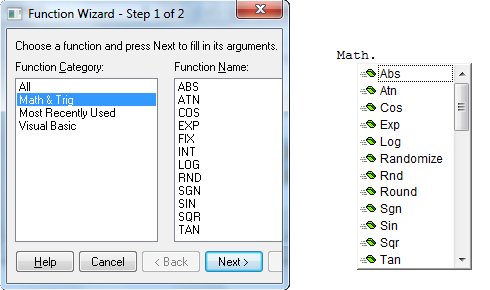The only important point to note are the SIN, COS and TAN functions which take the angle in radians and not degrees.

• Logical functions

These remain largely un-documented. Use of logical operators like Less than (<),  greater than (>), <= and >= are permitted in the formula for a Variable.

For example, a simple implementation of the =Min(V1,V2) function can be written as:
= -1*( V1 < V2 )* V1 +(-1*( V2 <= V1 )* V2)

In the above formula, the logical operator returns a -1 or 0 based on whether the condition evaluates to true or false. So given, V1 = 15 and V2 = 45, the above formula evaluates as follows:
= -1*(-1)* 15 + (-1 * (0) * 45)
= -15 + 0
= 15

This way the smaller number is always returned. Such type of formula are difficult to write and interpret hence rarely used.

• VBScript Functions and Sub-routines

Take an example of a shaft as shown below.
The various dimension variables are seen in the Variable Table.
The design of various parameters is as below:
1. Keyway length is always 75 less than the shaft length.
2. Hole diameter is 4.5 if ShaftDia is 20 or above and 2.75 if ShaftDia is less than 20.
3. Shaft diameter has predefined values and a corresponding diameter for the hole.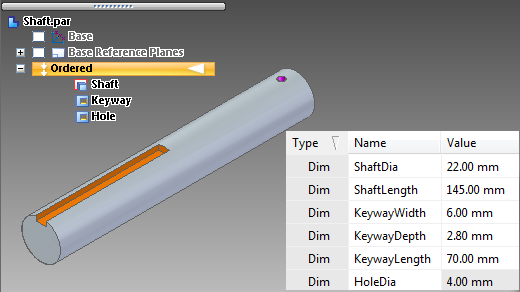Lets see the three cases in greater details.

Case 1. The keyway length can be set right into the variable table by specifying it as:
= (ShaftLength – 75)

Note: It is always advisable to enclose even the simplest of expressions in parentheses.

This also presents an opportunity to setup a simple VB function to calculate the value for the KeywayLength variable. Start notepad and save the file as Shaft.bas making sure it does not have the .txt in addition. Type in the following:

Function KeywayLength(ByVal ShaftLength As Double) As Double
KeywayLength = (ShaftLength – 75)
End Function

Notes:
• The keyword Function indicates beginning of function definition.
• The name of the function ‘KeywayLength’ can be same as the variable name that is  calculated.
• The variable ShaftLength from the variable table is provided to the function in the round brackets or parentheses.
• Double simply means a decimal number. For integer values, the word Integer is used.
• A variable KeywayLength which is same as the function name is then calculated using the ShaftLength provided.
• End Function denotes, the end of function definition.
• Also note the ‘As Double’ at the end of function declaration on line 1. The ‘As Double’ indicates that the KeywayLength is also calculated as a decimal number.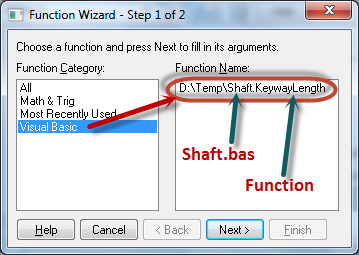How to use in Solid Edge:
• Click in the formula cell for the KeywayLength variable in the variable table.
• Click the Formula (Fx) button in the variable table.
• The Function Wizard dialog appears. Select Visual Basic in the list.
• Select the Shaft.bas file. The function KeywayLength appears in the list on the right side of the Wizard dialog. Select it.
• Click Next.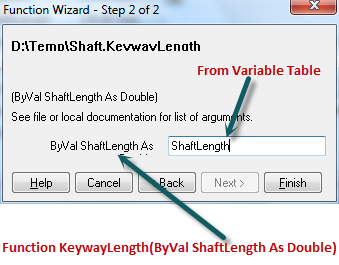• Step 2 of the wizard appears asking for the arguments for the function.
• Type ShaftLength as the argument. This ShaftLength from the Variable Table is provided to the ShaftLength variable of the function.
• Click Finish. The formula cell is filled with the path and name of the bas file and the function name with the argument ‘ShaftLength’ in brackets.
• Change the ShaftLength variable in the variable table and verify the KeywayLength updates accordingly.

Tip: Make sure the ‘Automatic Update’ button is checked ON on the Tools tab, Links group.

Case 2. Hole diameter is 4.5 if ShaftDia is above 20 and 2.75 if ShaftDia is less than 20.
For this add a function as below to the .bas file:

Function HoleDia(ByVal ShaftDia As Double) As Double
If ShaftDia >= 20 Then
HoleDia = 4.5
ElseIf ShaftDia < 20 Then
HoleDia = 2.75
End If
End Function

and the formula for the HoleDia variable updates as below:
D:TempShaft.HoleDia( ShaftDia )
Experiment by changing the ShaftDia value to 20, less than 20 and more than 20.

Case 3. Shaft diameter has predefined values and has a corresponding diameter for the hole as below: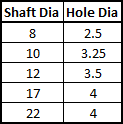The first step is to limit the values of the ShaftDia in the Variable Table to a range of given values. For this

• Right click the ShaftDia variable in the variable table and select Variable Rule Editor from the menu.
• Check ON “Limit Value To:”.
• Select the radio button for ‘Descrete List:’ and specify the range as:
{8.00 mm;10.00 mm;12.00 mm;17.00 mm;22.00 mm}
• The values are separated by a semi-colon and enclosed in curly braces. This automatically creates a drop-down list for the ShaftDia variable. Double click the current value to reveal the list.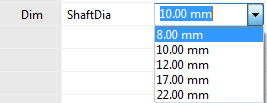To pick the value of HoleDia corresponding to the ShaftDia, write the function as below:

Function HoleDia(ByVal ShaftDia As Double) As Double
Select Case ShaftDia
Case 8
HoleDia = 2.5
Case 10
HoleDia = 3.25
Case 12
HoleDia = 3.5
Case 17
HoleDia = 4
Case 22
HoleDia = 4
End Select
End Function

Here, the Select Case structure helps to set a value for a variable corresponding to another variable passed to the function.

As mentioned earlier, there many more logical constructs in the BASIC language, thus presenting huge possibilites and complexity to user-defined functions. The techniques discussed here should be helpful to make a small beginning.

TOPICS
This article first appeared on the Siemens Digital Industries Software blog at https://blogs.sw.siemens.com/solidedge/vb-in-the-variable-table-skys-the-limit/